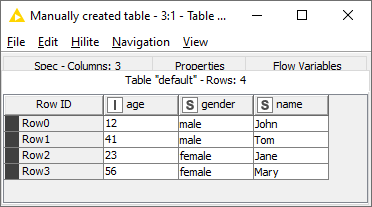# Column expressions node: columnNames() function does not return strings but numerical indexes

I am creating a Column Expressions node where I pass in both Flow Variables and a table. The variables have names identical to the columns in the table, e.g., “age”, “gender”, and contain a coefficient (number).

I want to create a sum:
sum = 0.0
for (each in columnNames()) {
sum = sum + variable(each)*column(each);
}

This fails with an error that variable(0) does not exist (which is true; variable(“age”) etc. do instead). Using a constant like 1.0 instead works perfectly.

On investigation I found that the columnNames() function does not provide the names, but the indexes instead, contrary to the documentation.

How to get the real column names? I cannot use numerical variable names, and a variableNames() function does not exist either.

Can you provide more information? Data and a workflow would be nice.

The columnNames() function does indeed provide an array of column names:The problem is the variable reference, because without it everything works:

It’s not clear to me how to use the iterative “each” in this case to retrieve the variables.

1 Like

Hi,
And many thanks for a very quick answer!

You are absolutely correct that the columnNames() function returns an array of column names, like you say.

My mistake was here:

sum = 0.0
for (each in columnNames()) {
sum = sum + variable(each)*column(each);
}

My assumption was that the variable each would be every name in turn (like Python). Turns out this is not the case; it will be the index instead. But the column(each) works fine because you can retrieve the columns using an index, but variable not.

sum = 0.0
for (each in columnNames()) {
name = columnNames()[each];
sum = sum + variable(name)*column(name);
}

which is just fine; or perhaps nicer-looking:

sum = 0.0
cols = columnNames()
for (each in cols) {
name = cols[each];
sum = sum + variable(name)*column(name);
}

Many thanks for making me think and for your patience!

Cheers,
Sam

1 Like

This topic was automatically closed 90 days after the last reply. New replies are no longer allowed.